#### Acceleration

The next step is to mathematically describe the change in velocity, which results in the acceleration,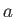; this is defined as: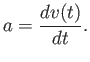(8.5)

The form is the same as (8.1), except that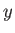has been replaced by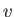. Approximations can similarly be made. For example,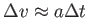.

The acceleration itself can vary over time, resulting in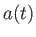. The following integral relates acceleration and velocity (compare to (8.4)):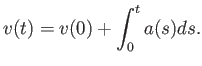(8.6)

Since acceleration may vary, you may wonder whether the naming process continues. It could, with the next derivative called jerk, followed by snap, crackle, and pop. In most cases, however, these higher-order derivatives are not necessary. One of the main reasons is that motions from classical physics are sufficiently characterized through forces and accelerations. For example, Newton's Second Law states that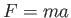, in which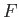is the force acting on a point,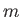is its mass, andis the acceleration.

For a simple example that should be familiar, consider acceleration due to gravity,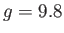m/s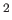. It is as if the ground were accelerating upward by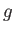; hence, the point accelerates downward relative to the Earth. Using (8.6) to integrate the acceleration, the velocity over time is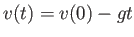. Using (8.4) to integrate the velocity and supposing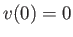, we obtain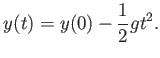(8.7)

Steven M LaValle 2020-11-11